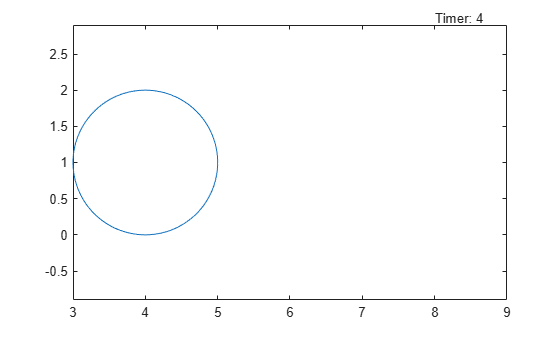Main Content

# rewindAnimation

Rewind previously played animation objects

## Syntax

``rewindAnimation``
``rewindAnimation(fig)``

## Description

example

````rewindAnimation` rewinds previously played animation objects by restoring the animation time parameter to its initial value. The animation objects must be created using the `fanimator` function.```

example

````rewindAnimation(fig)` rewinds animation objects in the figure `fig`.```

## Examples

collapse all

Create an animation of a moving circle and rewind it using `rewindAnimation`.

First, create two symbolic variables, `t` and `x`. The variable `t` defines the time parameter of the animation. Use `t` to set the center of the circle at `(t,1)` and `x` to parameterize the perimeter of the circle within the range `[-pi pi]`. Create the circle animation object using `fanimator`. Set the x-axis and y-axis to be equal length.

```syms t x fanimator(@fplot,cos(x)+t,sin(x)+1,[-pi pi]) axis equal```

Play the animation by entering the command `playAnimation`. By default, `playAnimation` plays the animation within the range of `t` from 0 to 10. You can rewind the animation by using `rewindAnimation`. `rewindAnimation` restores the animation time parameter to its initial value at `t = 0` and shows the starting animation frame.

`rewindAnimation`Create an animation of a moving circle with a timer, and rewind the animation using `rewindAnimation`.

First, create two symbolic variables, `t` and `x`. The variable `t` defines the time parameter of the animation. Create a figure window for the animation.

```syms t x fig = figure;```

Create the circle animation object using `fanimator`. Use `t` to set the center of the circle at `(t,1)` and `x` to parameterize the perimeter of the circle within the range `[-pi pi]`. Set the range of the animation time parameter to `[4 8]`. Set the x-axis and y-axis to be equal length.

```fanimator(@fplot,cos(x)+t,sin(x)+1,[-pi pi],'AnimationRange',[4 8]) axis equal```

Next, add a timer animation object. Use the `text` function to create a piece of text to count the elapsed time. Use `num2str` to convert the time parameter to a string.

```hold on fanimator(@(t) text(8,3,"Timer: "+num2str(t,2)),'AnimationRange',[4 8]) hold off```

Play the animation in figure `fig` between 4 and 8 seconds by entering the `playAnimation` command.

`playAnimation(fig,'AnimationRange',[4 8])`

You can rewind a previously played animation by using `rewindAnimation`. `rewindAnimation` restores the animation time parameter to its initial value at `t = 4` and shows the starting animation frame.

`rewindAnimation(fig)`## Input Arguments

collapse all

Target figure, specified as a `Figure` object. For more information about `Figure` objects, see `figure`.

## See Also

Introduced in R2019a

## Support

#### Mathematical Modeling with Symbolic Math Toolbox

Get examples and videos